#[資料結構] 使用 C 語言：以陣列 (Array) 為基礎的堆疊 (Stack)

## 堆疊的抽象資料結構

``````S is a stack.

sub IsEmpty(S): bool
(Optional) sub IsFull(S): bool
(Optional) sub Size(S): sz
sub Peek(S): data
sub Push(S, data): void
sub Pop(S): data``````

## 堆疊型態的公開界面

``````#ifndef STACK_H
#define STACK_H

#ifndef __cplusplus
#include <stdbool.h>
#endif

typedef struct stack_t stack_t;

#ifdef __cplusplus
extern "C" {
#endif

stack_t * stack_new(void);
void stack_delete(void *self);
bool stack_is_empty(const stack_t *self);
int stack_peek(const stack_t *self);
bool stack_push(stack_t *self, int data);
int stack_pop(stack_t *self);

#ifdef __cplusplus
}
#endif

#endif  /* STACK_H */``````

## 宣告堆疊類別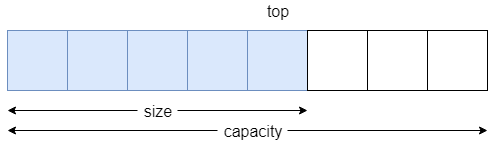``````typedef struct stack stack_t;

struct stack {
size_t size;
size_t capacity;
size_t top;
int *elements;
};``````

## 堆疊的建構函式

``````stack_t * stack_new(void)
{
stack_t *s = malloc(sizeof(stack_t));
if (!s)
return s;

s->size = 0;
s->capacity = 2;
s->top = 0;
s->elements = malloc(s->capacity * sizeof(int));
if (!(s->elements)) {
stack_delete(s);
s = NULL;
return s;
}

return s;
}``````

## 堆疊的解構函式

``````void stack_delete(void *self)
{
if (!self) {
return;
}

int *elements = ((stack_t *) self)->elements;
if (elements)
free(elements);

free(self);
}``````

## 檢查堆疊是否為空

``````bool stack_is_empty(const stack_t *self)
{
assert(self);
return self->size == 0;
}``````

## 檢視堆疊頂端的資料

``````int stack_peek(const stack_t *self)
{
assert(!stack_is_empty(self));

return self->elements[self->top];
}``````

## 將資料推入堆疊頂端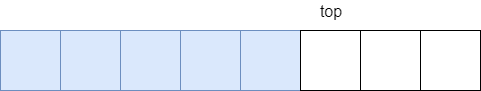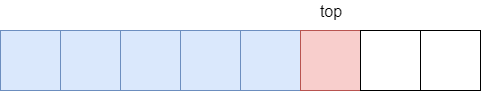``````bool stack_push(stack_t *self, int data)
{
if (!stack_expand(self))
return false;

if (stack_size(self) > 0)
self->top = (self->top + 1) % self->capacity;

self->elements[self->top] = data;
self->size++;

return true;
}``````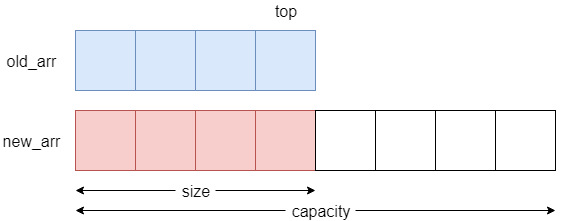``````static bool stack_expand(stack_t *self)
{
if (self->size < self->capacity) {
return true;
}

int *old_arr = self->elements;
self->capacity = self->size * 2;
int *new_arr = malloc(self->capacity * sizeof(int));
if (!new_arr) {
return false;
}

size_t sz = 0;
int i = self->top;
int j = self->top;
while (sz < self->capacity) {
new_arr[i] = old_arr[j];

i = i == 0 ? self->capacity - 1 : i - 1;
j = j == 0 ? self->size - 1 : j - 1;
sz++;
}

self->elements = new_arr;
free(old_arr);

return true;
}``````

## 將資料移出堆疊頂端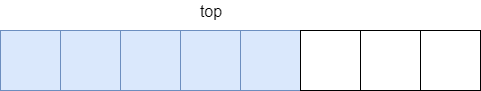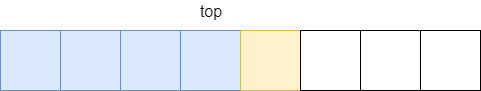``````int stack_pop(stack_t *self)
{
assert(!stack_is_empty(self));

int popped = self->elements[self->top];

self->top = self->top == 0 ? self->capacity - 1 : self->top - 1;
self->size--;

return popped;
}``````

## 演算法上的效率

• `IsEmpty(S)``O(1)`
• `Peek(S)``O(1)`
• `Push(S, data)`：amortized `O(1)`
• `Pop(S)``O(1)`

`Push` 在堆疊擴張時，程式效率為 `O(n)`，但不是每次推入元素時都要擴張堆疊，透過攤平分析法 (amortized analysis) 可知效率為約略為 `O(1)`